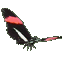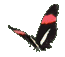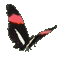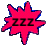Math Help - Subtract Fractions - Game Tips:

- The fraction 2/3 can be subtracted from 5/6 by first converting 2/3 into 4/6 .
Then you do the subtraction (5/6)-(4/6) to get the answer 1/6 .

- In order to subtract two fractions, you convert them to the same denominator (common denominator).
- You can convert a fraction by multiplying the top and bottom by the same number.

In the above example, 2/3 = (2x2)/(3x2) = 4/6.

- Another example: 5/6 - 1/4 = (5x4)/(6x4) - (6x1)/(6x4) = 20/24 - 6/24 = 14/24 which reduces to 7/12.

- The general rule is   a/b - c/d = (ad-bc)/(bd) .

- Single denominators used in this game are less than 20. (Product denominators<300).

- Your Score Report appears after you have made 6 choices.
- Your Game Score is reduced by the number of butterfly hits.

- To slow the game speed repeat tap/click on the word Slider.
- To increase the game speed repeat tap/click on the word Math.
- Speed can also be adjusted with a keyboard's - and + keys.

- Refresh/Reload the web page to restart the game.
- Adjust the sound level of media on your device.

More SliderMath